# Overview of Conditional Random Fields

Conditional Random Fields are a discriminative model, used for predicting sequences. They use contextual information from previous labels, thus increasing the amount of information the model has to make a good prediction. In this post, I will go over some topics that will introduce CRFs. I will go over:

1. What Discriminative classifiers are (and how they compare to Generative classifiers)
2. Mathematical overview of Conditional Random Fields
3. How CRFs differ from Hidden Markov Models
4. Applications of CRFs

# What Discriminative classifiers are

Machine Learning models have two common categorizations, Generative and Discriminative. Conditional Random Fields are a type of Discriminative classifier, and as such, they model the decision boundary between the different classes. Generative models, on the other hand, model how the data was generated, which after having learnt, can be used to make classifications. As a simple example, Naive Bayes, a very simple and popular probabilistic classifier, is a Generative algorithm, and Logistic Regression, which is a classifier based on Maximum Liklihood estimation, is a discriminative model. Let’s look at how these models can be used to calculate label predictions:

Naive Bayes classifier is based on the Naive Bayes algorithm, which states the following:

The prediction we are trying to make with the classifier can be represented as a conditional probability, which we can use the Naive Bayes algorithm to decompose: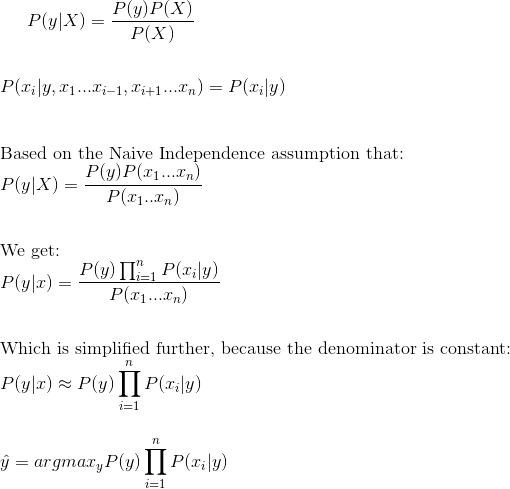Derivation of the Naive Bayes classifier

Logistic Regression classifier is based on the Logistic function, known as follows:

To learn the decision boundary between the two classes in Logistic Regression, the classifier learns weights associated with each data point (Theta values), and written as follows: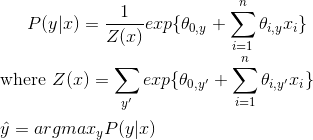Derivation of the Logistic Regression Classifier

With the Logistic Regression classifier, we see that we are maximizing the conditional probability, which by applying the Bayes rule, we can obtain the Generative classifier that is used by the Naive Bayes classifier.

We replace P(y | x) with the bayes equation: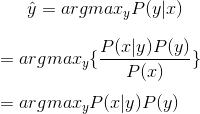Applying Bayes Rule to Logistic Regression Classifier

and set it equivalent to the product of the prior and the liklihood, since in an arg-max, the denominator P(x) does not contribute any information.

The result is the Generative classifier obtained earlier for Naive Bayes algorithm.

We can further see that P(x | y) * P(y) is equal to P(x, y), the joint distribution of x and y. This observation supports the earlier definition of Generative classifiers. By modeling the joint probability distribution between the classes, the Generative model can be used to obtain and “generate” the input points X, given the label Y and the joint probabality distribution. Similarly, the discriminative model, by learning the conditional probability distribution, has learnt the decision boundary that separates the data points. So, given an input point, it can use the conditional probabality distribution to calculate it’s class.

How do these definitions apply to Conditional Random Fields? Conditional Random Fields are a Discriminative model, and their underlying principle is that they apply Logistic Regression on sequential inputs. If you are familiar with Hidden Markov Models, you will find that they share some similarities with CRFs, one in that they are also used for sequential inputs. HMMs use a transition matrix and the input vectors to learn the emission matrix, and are similar in concept to Naive Bayes. HMMs are a Generative model.

# Mathematical overview of Conditional Random Fields

Having discussed the above definitions, we will now go over Conditional Random Fields, and how they can be used to learn sequential data.

As we showed in the previous section, we model the Conditional Distribution as follows:

In CRFs, our input data is sequential, and we have to take previous context into account when making predictions on a data point. To model this behavior, we will use Feature Functions, that will have multiple input values, which are going to be:

1. The set of input vectors, X
2. The position i of the data point we are predicting
3. The label of data point i-1 in X
4. The label of data point i in X

We define the feature function as:

The purpose of the feature function is to express some kind of characteristic of the sequence that the data point represents. For instance, if we are using CRFs for Parts-of-Speach tagging, than

f (X, i, L{i - 1}, L{i} ) = 1 if L{i - 1} is a Noun, and L{i} is a Verb. 0 otherwise.

Similarly, f (X, i, L{i - 1}, L{i} ) = 1 if L{i - 1} is a Verb and L{i} is an Adverb. 0 otherwise.

Each feature function is based on the label of the previous word and the current word, and is either a 0 or a 1. To build the conditional field, we next assign each feature function a set of weights (lambda values), which the algorithm is going to learn: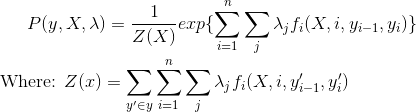Probability Distribution for Conditional Random Fields

To estimate the parameters (lambda), we will use Maximum Liklihood Estimation. To apply the technique, we will first take the Negative Log of the distribution, to make the partial derivative easier to calculate: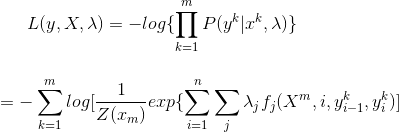Negative Log Liklihood of the CRF Probability Distribution

To apply Maximum Liklihood on the Negative Log function, we will take the argmin (because minimizing the negative will yield the maximum). To find the minimum, we can take the partial derivative with respect to lambda, and get:

We use the Partial Derivative as a step in Gradient Descent. Gradient Descent updates parameter values iteratively, with a small step, until the values converge. Our final Gradient Descent update equation for CRF is:

As a summary, we use Conditional Random Fields by first defining the feature functions needed, initializing the weights to random values, and then applying Gradient Descent iteratively until the parameter values (in this case, lambda) converge. We can see that CRFs are similar to Logistic Regression, since they use the Conditional Probability distribution, but we extend the algorithm by applying Feature functions as our sequential inputs.

# How CRFs differ from Hidden Markov Models

From the previous sections, it must be obvious how Conditional Random Fields differ from Hidden Markov Models. Although both are used to model sequential data, they are different algorithms.

Hidden Markov Models are generative, and give output by modeling the joint probability distribution. On the other hand, Conditional Random Fields are discriminative, and model the conditional probability distribution. CRFs don’t rely on the independence assumption (that the labels are independent of each other), and avoid label bias. One way to look at it is that Hidden Markov Models are a very specific case of Conditional Random Fields, with constant transition probabilities used instead. HMMs are based on Naive Bayes, which we say can be derived from Logistic Regression, from which CRFs are derived.

# Applications of CRFs

Given their ability to model sequential data, CRFs are often used in Natural Language Processing, and have many applications in that area. One such application we discussed is Parts-of-Speech tagging. Parts of speech of a sentence rely on previous words, and by using feature functions that take advantage of this, we can use CRFs to learn how to distinguish which words of a sentence correspond to which POS. Another similar application is Named Entity recognition, or extracting Proper nouns from sentences. Conditional Random Fields can be used to predict any sequence in which multiple variables depend on each other. Other applications include parts-recognition in Images and gene prediction.

To read more about Conditional Random Fields and other topics discussed in this post, refer to the following links:

https://stackoverflow.com/questions/879432/what-is-the-difference-between-a-generative-and-discriminative-algorithm [There are several answers in this post that provide a good overview of Discriminative and Generative algorithms.]

https://www.cs.cmu.edu/~tom/mlbook/NBayesLogReg.pdf [A detailed review between the differences in Naive Bayes and Logistic Regression.]

http://homepages.inf.ed.ac.uk/csutton/publications/crftut-fnt.pdf [Section 2.2 on Discriminative and Generative classifiers, and how they apply to Naive Bayes vs Logistic Regression.]

https://prateekvjoshi.com/2013/02/23/why-do-we-need-conditional-random-fields/ and https://prateekvjoshi.com/2013/02/23/what-are-conditional-random-fields/ [These are both very good posts on introduction to Conditional Random Fields and how they differ from graphical models (like HMMs)]

http://blog.echen.me/2012/01/03/introduction-to-conditional-random-fields/ [A light overview of Conditional Random Fields.]

http://www.lsi.upc.edu/~aquattoni/AllMyPapers/crf_tutorial_talk.pdf [Slide deck that goes over Conditional Random Fields, how to define feature functions, and steps on it’s derivation.]

--

--